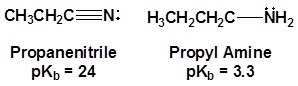# 20.1: Structure and Physical Properties of Acid Derivatives

$$\newcommand{\vecs}{\overset { \rightharpoonup} {\mathbf{#1}} }$$ $$\newcommand{\vecd}{\overset{-\!-\!\rightharpoonup}{\vphantom{a}\smash {#1}}}$$$$\newcommand{\id}{\mathrm{id}}$$ $$\newcommand{\Span}{\mathrm{span}}$$ $$\newcommand{\kernel}{\mathrm{null}\,}$$ $$\newcommand{\range}{\mathrm{range}\,}$$ $$\newcommand{\RealPart}{\mathrm{Re}}$$ $$\newcommand{\ImaginaryPart}{\mathrm{Im}}$$ $$\newcommand{\Argument}{\mathrm{Arg}}$$ $$\newcommand{\norm}{\| #1 \|}$$ $$\newcommand{\inner}{\langle #1, #2 \rangle}$$ $$\newcommand{\Span}{\mathrm{span}}$$ $$\newcommand{\id}{\mathrm{id}}$$ $$\newcommand{\Span}{\mathrm{span}}$$ $$\newcommand{\kernel}{\mathrm{null}\,}$$ $$\newcommand{\range}{\mathrm{range}\,}$$ $$\newcommand{\RealPart}{\mathrm{Re}}$$ $$\newcommand{\ImaginaryPart}{\mathrm{Im}}$$ $$\newcommand{\Argument}{\mathrm{Arg}}$$ $$\newcommand{\norm}{\| #1 \|}$$ $$\newcommand{\inner}{\langle #1, #2 \rangle}$$ $$\newcommand{\Span}{\mathrm{span}}$$$$\newcommand{\AA}{\unicode[.8,0]{x212B}}$$

## The Structure of Carboxylic Acid Derivatives

Carboxylic acid and its derivatives are functional groups with closely related chemistry.The carboxylic acid derivatives all include an acyl group, an R-group bonded to a carbonyl carbon. The "other group" bonded to the carbonyl carbon distinguishes the derivatives from each other and has a strong influence on the relative reactivity between the derivatives. This "other group" takes the role of the "Leaving Group or LG" in Nucleophilic Acyl Substitution (NAS) reactions.Although there are many types of carboxylic acid derivatives known, this chapter focuses on four: acid halides (acyl halides), acid anhydrides, esters, and amides. Another common feature of carboxylic acid derivatives is that they can be hydrolyzed back to the original carboxylic acid. For this reason, nitriles are sometimes grouped with the carboxylic acid derivatives and will also be discussed in this chapter. The hydrolysis reaction for acetonitrile is shown below as an example.## Physical Properties of Carboxylic Acid Derivatives

The intermolecular forces of the respective acyl group combine with the size and structure of the R-group to determine the physical properties of the the carboxylic acid derivatives as shown in the summary below.## Structure and Properties of Nitriles

The electronic structure of nitriles is very similar to that of an alkyne with the main difference being the presence of a set of lone pair electrons on the nitrogen. Both the carbon and the nitrogen are sp hydridized which leaves them both with two p orbitals which overlap to form the two $$\pi$$ bond in the triple bond. The R-C-N bond angle in and nitrile is 180o which give a nitrile functional group a linear shape.The lone pair electrons on the nitrogen are contained in a sp hybrid orbital which makes them much less basic and an amine. The 50% character of an sp hybrid orbital close to the nucleus and therefore less basic compared to other nitrogen containing compounds such as amines.The presence of an electronegative nitrogen causes nitriles to be very polar molecules. Consequently, nitriles tend to have higher boiling points than molecules with a similar size.Exercise

1. Design an extraction separation strategy to separate acetaminophen from cocaine using ether, 1 M HCl, and 3 M NaHCO3. The structures for acetaminophen and cocaine are shown below.